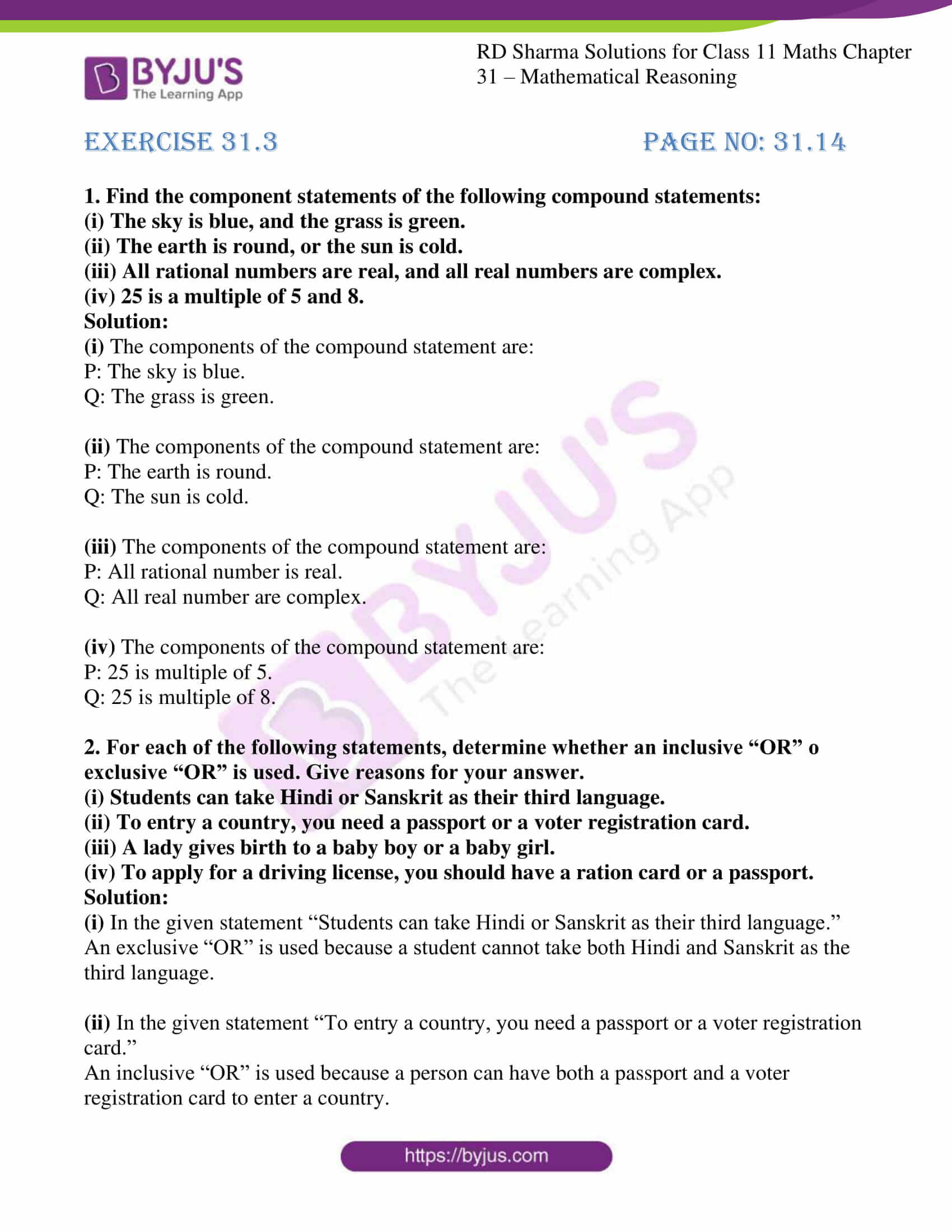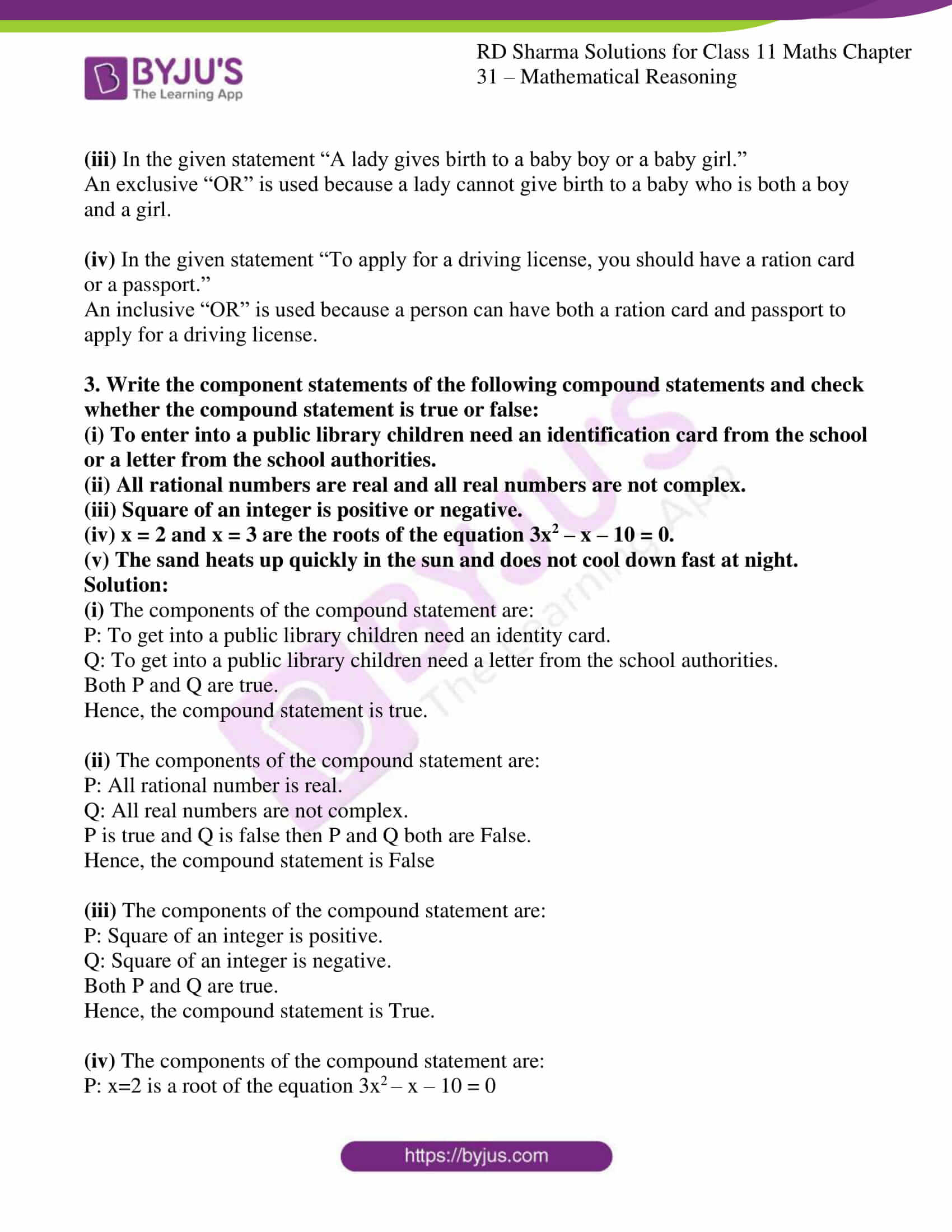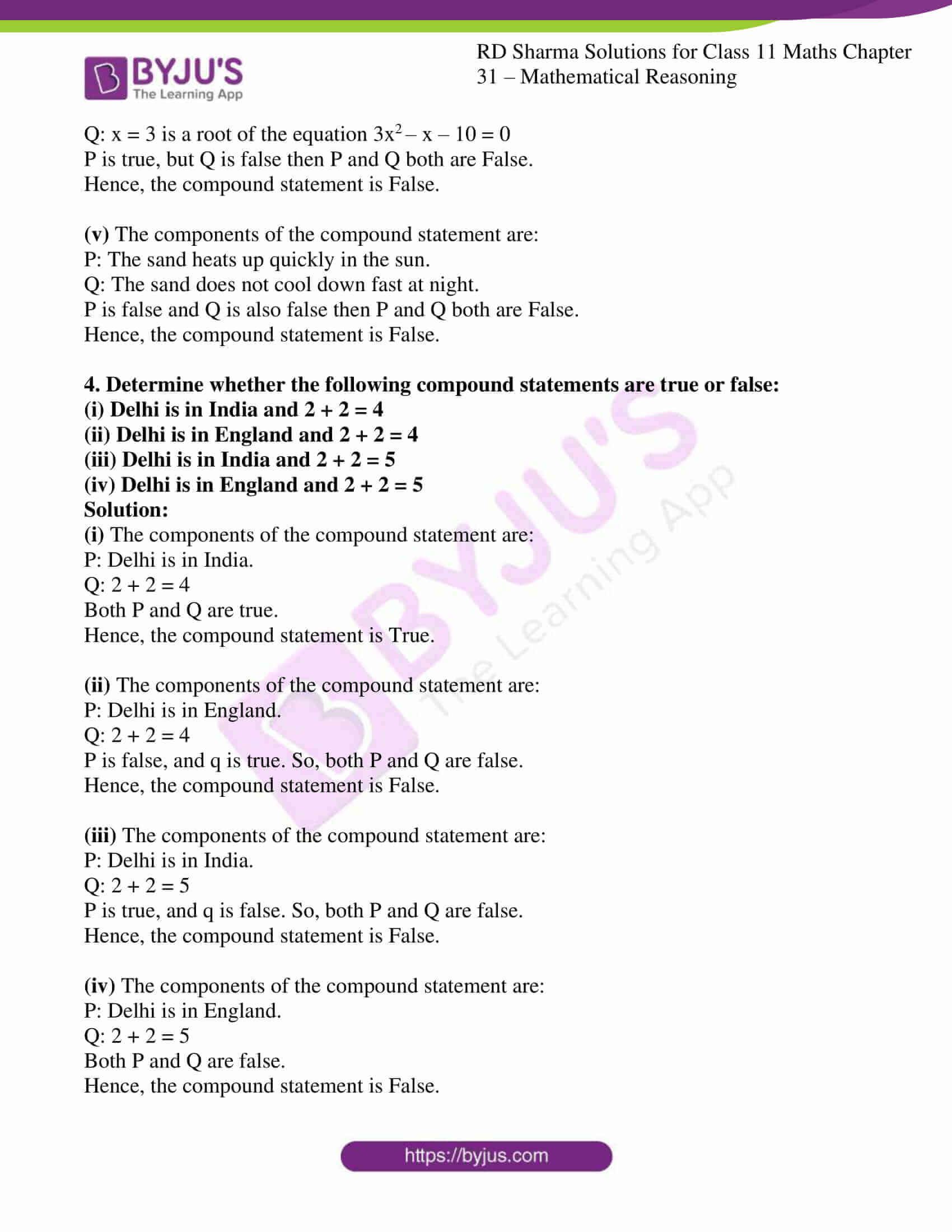# RD Sharma Solutions for Class 11 Chapter 31 - Mathematical Reasoning Exercise 31.3

In this section, we shall discuss compound statements and basic connectives such as ‘AND’ and ‘OR’. “if a statement is a combination of two or more simple statements, it is termed as compound statements”. The solutions have been prepared by our expert faculty team to help students analyse their weaker sections and work on it, to yield good results. RD Sharma Class 11 Maths Solutions is one of the best reference guides that students can rely on and also help build their confidence in solving problems. It also provides strong conceptual knowledge among students to explore the key points in presenting a solution to a problem. Students can download the free pdf of RD Sharma solutions from the below mentioned links.

## Download the pdf of RD Sharma Solutions for Class 11 Maths Exercise 31.3 Chapter 31 – Mathematical Reasoning### Access answers to RD Sharma Solutions for Class 11 Maths Exercise 31.3 Chapter 31 – Mathematical Reasoning

#### EXERCISE 31.3 PAGE NO: 31.14

1. Find the component statements of the following compound statements:
(i) The sky is blue, and the grass is green.
(ii) The earth is round, or the sun is cold.
(iii) All rational numbers are real, and all real numbers are complex.
(iv) 25 is a multiple of 5 and 8.

Solution:

(i) The components of the compound statement are:

P: The sky is blue.

Q: The grass is green.

(ii) The components of the compound statement are:

P: The earth is round.

Q: The sun is cold.

(iii) The components of the compound statement are:

P: All rational number is real.

Q: All real number are complex.

(iv) The components of the compound statement are:

P: 25 is multiple of 5.

Q: 25 is multiple of 8.

2. For each of the following statements, determine whether an inclusive “OR” o exclusive “OR” is used. Give reasons for your answer.
(i) Students can take Hindi or Sanskrit as their third language.
(ii) To entry a country, you need a passport or a voter registration card.
(iii) A lady gives birth to a baby boy or a baby girl.
(iv) To apply for a driving license, you should have a ration card or a passport.

Solution:

(i) In the given statement “Students can take Hindi or Sanskrit as their third language.”

An exclusive “OR” is used because a student cannot take both Hindi and Sanskrit as the third language.

(ii) In the given statement “To entry a country, you need a passport or a voter registration card.”

An inclusive “OR” is used because a person can have both a passport and a voter registration card to enter a country.

(iii) In the given statement “A lady gives birth to a baby boy or a baby girl.”

An exclusive “OR” is used because a lady cannot give birth to a baby who is both a boy and a girl.

(iv) In the given statement “To apply for a driving license, you should have a ration card or a passport.”

An inclusive “OR” is used because a person can have both a ration card and passport to apply for a driving license.

3. Write the component statements of the following compound statements and check whether the compound statement is true or false:
(i) To enter into a public library children need an identification card from the school or a letter from the school authorities.
(ii) All rational numbers are real and all real numbers are not complex.
(iii) Square of an integer is positive or negative.
(iv) x = 2 and x = 3 are the roots of the equation 3x2 – x – 10 = 0.
(v) The sand heats up quickly in the sun and does not cool down fast at night.

Solution:

(i) The components of the compound statement are:

P: To get into a public library children need an identity card.

Q: To get into a public library children need a letter from the school authorities.

Both P and Q are true.

Hence, the compound statement is true.

(ii) The components of the compound statement are:

P: All rational number is real.

Q: All real numbers are not complex.

P is true and Q is false then P and Q both are False.

Hence, the compound statement is False

(iii) The components of the compound statement are:

P: Square of an integer is positive.

Q: Square of an integer is negative.

Both P and Q are true.

Hence, the compound statement is True.

(iv) The components of the compound statement are:

P: x=2 is a root of the equation 3x2 – x – 10 = 0

Q: x = 3 is a root of the equation 3x2 – x – 10 = 0

P is true, but Q is false then P and Q both are False.

Hence, the compound statement is False.

(v) The components of the compound statement are:

P: The sand heats up quickly in the sun.

Q: The sand does not cool down fast at night.

P is false and Q is also false then P and Q both are False.

Hence, the compound statement is False.

4. Determine whether the following compound statements are true or false:
(i) Delhi is in India and 2 + 2 = 4
(ii) Delhi is in England and 2 + 2 = 4
(iii) Delhi is in India and 2 + 2 = 5
(iv) Delhi is in England and 2 + 2 = 5

Solution:

(i) The components of the compound statement are:

P: Delhi is in India.

Q: 2 + 2 = 4

Both P and Q are true.

Hence, the compound statement is True.

(ii) The components of the compound statement are:

P: Delhi is in England.

Q: 2 + 2 = 4

P is false, and q is true. So, both P and Q are false.

Hence, the compound statement is False.

(iii) The components of the compound statement are:

P: Delhi is in India.

Q: 2 + 2 = 5

P is true, and q is false. So, both P and Q are false.

Hence, the compound statement is False.

(iv) The components of the compound statement are:

P: Delhi is in England.

Q: 2 + 2 = 5

Both P and Q are false.

Hence, the compound statement is False.